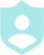# Solving Quadratic Equations by Factoring###### Example QuestionsSolve each equation by factoring.Solve each equation by factoring.
##### Description :
Practice solving quadratic equations by factoring.
##### Difficulty :TRIVIA 10 QuestionsDownloadPrintShareAdd
Questions

•Solve each equation by factoring.Colors :
Font color
Button color
Background color
Logo:Background Image:Theme Song: